## Saturday 21 November 2015

### Maximum Beam Spans

Beams are structural elements (which are typically horizontal or nearly horizontal) that span between supports to carry gravity loads over a space below the beam. In designing a beam for strength, it is necessary to check resistance to bending and shear stresses. It may also be necessary to check bearing stresses at supports.

When designing a beam, it is also necessary to ensure that the deflections are acceptable. While a flexible beam might have adequate strength, excessive deflections can damage finishes like ceiling drywall and floor tiles. Floor beams may need to be checked for vibrations as well. Excessive floor vibrations due to the activities of the occupants (e.g. walking, dancing, etc.) perceived by people in the building might make them uncomfortable. Some degree of rigidity and vibration resistance is required in order for the general public to feel confident in the structure's safety.

In order to calculate deflections or the structural demand of a beam, it is necessary to know the applied load and the beam span. That's fine if you're evaluating the strength of an existing beam or if you're sizing beams based on where the architect has allowed you to have columns or load-bearing walls. In these cases the span of the beam has been determined and a the engineer needs to size the beam to safely carry the design loads. But what if, for whatever reason, you already have the size of the beam and want to know how far apart you can place supports? This scenario might be encountered in a preliminary design stage, especially if you're attempting to develop an economical design using standard material sizes and spacing. For example, perhaps you want to minimize the cost per square foot of a wooden deck constructed using 2x8 lumber.

Calculating maximum spans might start to get cumbersome if you've got to consider multiple load patterns and failure modes. However, there are many scenarios where you can simplify things enough that having a maximum span table makes sense, such as in wood floor design. In this post I derive some formulas that can be used to calculate maximum beam spans. I have assumed that the beams are supported at the ends only, the ends are free to rotate about the axes perpendicular to the length of the beam, and the beams are subjected to a uniformly distributed load. The shortest span calculated after checking all possible failure modes would be the maximum permissible span.

Failure Mode: Shear strength

The demand on the beam is the maximum applied shear force, Vf. For a beam with uniformly distributed load w, the maximum applied shear force is:

A beam's maximum resistance to shear, Vr, is the product of some allowable shear stress and the beam's effective shear area. In stocky beams, the allowable shear stress depends only on the strength of the material that the beam is made from. In thin-walled beams, allowable shear stress may be reduced due to buckling, in which case the maximum shear stress is a function of other factors, such as the depth-to-thickness ratio of the beam web.

The beam's resistance to shear failure must exceed the demand in the beam (Vr > Vf). Therefore, the maximum beam span for shear-controlled failure is:Maximum beam span for shear-controlled failure

Failure Mode: Bearing strength

The demand on the bearing is the maximum support reaction force. Since the beam is supported only at either end, the maximum support reaction is also equal to the maximum shear force. Bearing resistance is determined by the bearing contact area multiplied by some allowable bearing stress, which could be limited by the strength of either the beam or the support material.

Fcp is the allowable stress in compression perpendicular to the beam and Ab is the area of bearing.
Solving for L, we get an expression that looks similar to the one for shear failure:Maximum beam span for bearing-controlled failure

Failure Mode: Bending strength

The demand on the beam is the maximum applied bending moment, Mf. For a beam with uniformly distributed load, the maximum applied bending moment is:

A beam's maximum resistance to bending, Mr, is the product of some allowable bending stress and a property of the cross-section known as the section modulus, S. In stocky beams, the allowable bending stress depends only on the strength of the material that the beam is made from. In thin-walled beams, allowable bending stress may be reduced due to distortional or local buckling effects. The spacing of lateral supports keeping the beam aligned can also reduce the allowable stress due to the effect of lateral-torsional buckling.

The beam's resistance to bending failure must exceed the demand in the beam (Mr > Mf). Therefore, the maximum beam span for bending-controlled failure is: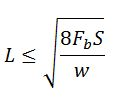Maximum beam span for bending-controlled failure

Failure Mode: Excessive absolute deflection

The deflection of a beam under uniform load is typically calculated from:

where E is the modulus of elasticity and I is the moment of inertia (second moment of area). Therefore, the maximum beam span to ensure the deflection does not exceed the maximum permissible value is calculated from: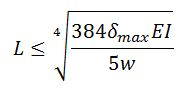Maximum span for deflection control

This was based on the assumption that the deflections of the beam come almost entirely from bending deformations. However, shear deformations may also need to be considered in cases where the beam's span to depth ratio is low and/or if the shear rigidity of the beam is small relative to the bending rigidity. A more precise formula to calculate the deflection of a beam under uniform load is:

where k is an empirical correction factor, A is the cross-sectional area, and G is the shear modulus. Research has shown that k is dependent on both the cross-sectional shape of the beam and Poisson's ratio of the material.

Solving for L here requires you to solve for the roots of a quartic equation. But there are no cubic or linear terms here, so we can treat it as quadratic, which you might remember is something you learned to solve in high school. So, based on the more accurate deflection formula, the maximum beam span to ensure the deflection does not exceed the maximum permissible value is:Maximum span for deflection control (including deflection due to shear deformations)

Failure Mode: Excessive deflection-to-span ratio

Instead of an absolute deflection limit, designers often choose a limiting ratio of deflection to beam span. A commonly selected maximum deflection is the span divided by 360. The span divisor, which I'll call SD, might be decreased to say 180 where the structure is not supporting anything sensitive to deflections, or it might be increased to say 800 or more where deflection-sensitive equipment is supported.  The deflection of a beam under uniform load is typically calculated from:

Therefore, the maximum beam span to ensure the deflection does not exceed the maximum permissible deflection-to-span ratio is calculated from:Maximum span for deflection control, based on limiting deflection/span ratio

Again, this was based on the assumption that the deflections of the beam come almost entirely from bending deformations. A more precise formula to calculate the deflection of a beam under uniform load is:

Solving for L here requires you to solve for the roots of a cubic equation. There's a closed form solution for that, and since there's no squared term in the expression, it's not too scary-looking. So, based on the more accurate deflection formula, the maximum beam span to ensure the deflection does not exceed the maximum permissible deflection-to-span ratio is:Maximum span for deflection control, based on limiting deflection/span ratio (including shear deformations)

So what does all this mean? Well, shear and bearing are proportional to span, bending moment is proportional to span squared, and deflection is a 4th order function of span. This means that for sufficiently large values of L, deflection will govern the design. Put another way, for very lightly loaded beams, the maximum span is a deflection-controlled span. For small values of L, shear or bearing may govern the design. Equivalently, for very heavily loaded beams, the maximum span might be controlled by shear or bearing strength. Here's an example with a steel bar, 2 inches wide by 4 inches deep. I've assumed a grade of steel with 44,000 psi yield strength, E = 29,000,000 psi, and G = 11,500,000 psi. I calculated allowable bending and shear stresses in accordance with the allowable stress design equations in AISC 360-2010, assuming the bar is laterally restrained at the supported ends only. I've also assumed that bearing strength is not an issue and that deflection must be less than L/180 or 2 inches, whichever is less.Maximum span versus applied load for a 2" by 4" steel beam.
In my example, deflections govern the maximum span for loads less that 3,350 lbs/ft). The beam has a self-weight of 27.2 lbs/ft, so the maximum permissible span based on the self-weight and the deflection limits is just over 380 inches (31'-8"). For practical beam spans, bending strength governs the span at loads greater than 3,350 lbs/ft. Shear theoretically wouldn't govern in this example until the applied load was about 451,000 lbs/ft, where the beam span would be just 6.7 inches. However, the beam theory used in this analysis would not be applicable for such a short span relative to the cross-sectional dimensions. (A 3D solid mechanics approach would be needed to accurately determine the capacity at very short spans). It would likely also be inappropriate to assume bearing stresses were not an issue for such a heavily loaded beam.

## Saturday 14 November 2015

### Dissecting the Pragmatic Speeder's Rationale

Drivers typically speed for one of two reasons: they derive pleasure from going fast, or they want to arrive at their destination sooner. In this post I analyze how effective speeding is at "making up time".

There's no question that driving faster will shorten your trip time, assuming you don't end up in an accident or stopped by a cop to be handed a speeding ticket because of it. Well, how much time do you actually save?

Let's say you're traveling a distance D down a highway (no stops along the way) where the posted speed limit is Vo. We'll call the duration of the trip at the speed limit to, which would be calculated from Equation 1.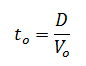Equation 1: Duration of trip if driven at the posted speed limit

Now you're thinking to yourself that you're not actually going to drive the speed limit, but some other speed V. We'll bring in another quantity, your speed ratio, alpha, which is simply V divided by Vo. If you're speeding, alpha > 1. If you're driving below the speed limit (say due to traffic or weather), alpha < 1.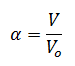Equation 2: Ratio of actual speed to posted speed limit

So the actual amount time your trip will take at velocity V is t, calculated from Equation 3 below.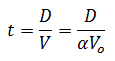Equation 3: Actual duration of trip

From here we can calculate how deviating from the posted speed limit will affect trip duration, whether you're speeding in an effort to make up time or trying to figure out how much extra time you'll need when you're driving through a snowstorm.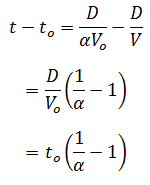Equation 4: Difference between actual duration and minimum legal duration

So if you want to cut your trip duration by just 10%, you need to drive 11.1% faster than the posted speed limit. A 10% cut in trip duration is only a difference of 6 minutes per hour. In a lot of places you can get away with 15 to 20% over the limit without drawing the attention of police, but even at 20% over you only cut the trip duration by 16.7% (10 minutes per hour). Not really an appreciable amount of time for typical trips. Say you left the house for work late and are trying to make up 10 minutes on a 30 minute commute. In that case you'd need to increase your average speed by 50%! Good way to draw the attention of police, not to mention the several-fold increase to your risk of involvement in a fatal collision. Here's a graph and table to show you how your speed ratio affects your trip duration.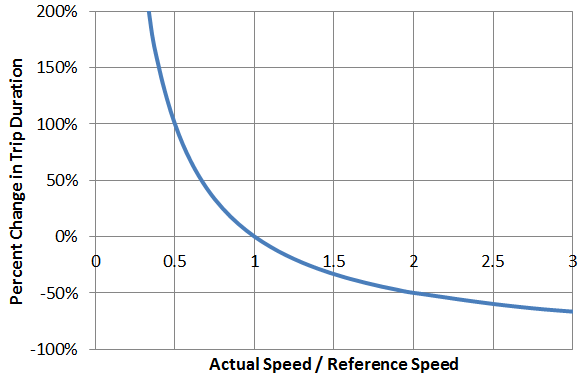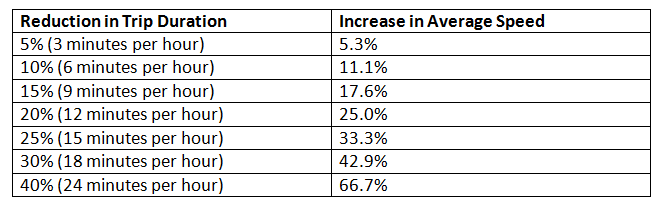To summarize, speeding a little bit saves you an insignificant amount of time, but in order to make up an appreciable amount of time you need to drive like a maniac. If you consider city driving instead of highway driving, the effect of speeding is even less significant because you spend so much time stopped at red lights. So, while it is technically true that speeding shortens your trip duration, the savings are hardly worth the added risks or costs associated with speeding, such as significantly increased likelihood of involvement in a fatal collision, wasted fuel, speeding fines, and higher insurance premiums.

## Sunday 1 November 2015

### SQA Higher Mathematics Examination 2015 (Part One)

This past year, the Scottish Qualifications Authority rolled out a new Higher Mathematics Examination, a test many high school students take to demonstrate they have an acceptable grasp of mathematics to be admitted to university. There are similar examinations on other subjects and Scottish universities typically require that you've taken and passed at least three of these Higher Examinations.

What's surprising about the 2015 examination is how poorly students performed on the test. A C-grade pass was awarded to students who scored as little as 34% on the test, and just 60% was need to receive an A-grade. Does someone who gets barely more than a third of the math right really represent the average student's skill level in mathematics? That's kind of a troubling thought.... When I heard about the low passing scores, I thought I'd try my hand at the test to see if it really was especially difficult.

Spoiler: I didn't finish. Read on to find out which question got the better of me.

In this post I review Part 1 of the examination paper and provide worked solutions. This portion of the test consisted of 15 questions, worth a total of 60 marks, to be solved without a calculator and within a 70 minute time limit. It means you need to know your stuff to finish on time, because you've got only an average of 4 minutes 40 seconds per question, or 1 minute 10 seconds per mark. Sitting at the desk idly thinking about how to solve a particular problem would be a sure way to score poorly on this test.

Problem 1

I thought this was a nice easy question to get the test started. The thing the student needed to recall here was that the dot product of two perpendicular vectors is equal to zero.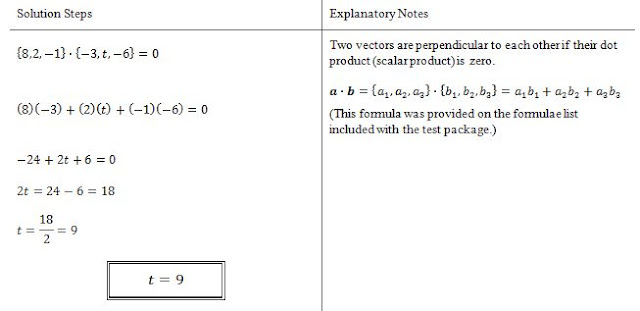Solution to Problem 1

Problem 2

I thought this was also fairly easy. Here the student needed to remember how to define the equation of a line, how to find the derivative of a polynomial, and that the slope of the tangent is equal to the derivative of the function. Taking the derivative of this expression is rudimentary calculus that shouldn't be a problem for most high school students.Solution to Problem 2

Problem 3

This one could cost you precious time if you didn't remember the Factor Theorem. That said, high school students who've just recently finished their math courses and have studied for their qualifications exams ought to have known about the Factor Theorem. Using the Factor Theorem, I solved this in about 3:24. A less efficient way to solve this would have involved polynomial division. I estimated that it would've taken me about 5:40 to solve it that way. At nearly 85 seconds per mark, that's a fair bit slower than the 70 seconds per mark average you need to maintain in order to finish the test. However, I used long division and I'm a little out of practice. I think a high school student who's still got synthetic division fresh in her mind might still be able solve this problem in under 70 seconds per mark.Solution to Problem 3

Problem 4

I didn't find this question very difficult either. Here you needed to recognize that the diagram shows a transformation of the cosine function, involving a vertical shift and both vertical and horizontal dilatation. It requires an understanding of how the terms p, q, and r affect the graph.Solution to Problem 4

Problem 5

This was definitely a fluff question. Inverting a linear function should be no problem for someone who just finished their high school mathematics courses.Solution to Problem 5

Problem 6

This question wasn't too difficult, as long as you remembered your laws of logarithms. But don't be deceived by the conciseness of the problem statement. If you didn't recognize which laws to use or how to factorize the numbers inside the log function, you could easily waste some time here.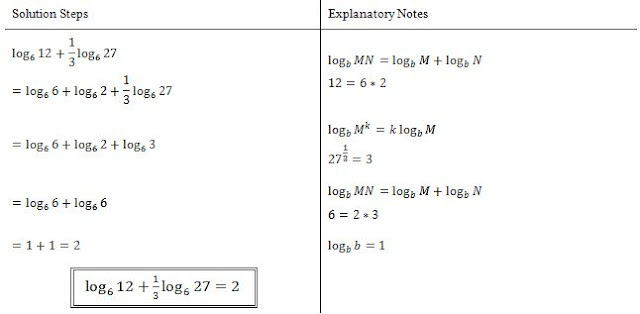Solution to Problem 6

Problem 7

Here's a problem that could really waste some time. The student needed to find the derivative of the function, but in order to solve this quickly it was also necessary to recognize that this function would be a lot easier to differentiate if it was expanded first. If you didn't expand, you'd be putting your high school calculus skills to the test, but also wasting time using the product rule, chain rule, and quotient rule all in this one little problem. I wouldn't be surprised if a significant proportion of students wasted time on this one by just ploughing ahead with chain rules and product rules.Solution to Problem 7

Problem 8
 The algebra on this one is pretty easy, but I can see how a student could easily lose a few marks here. First, you have to recall that the area of a rectangle is length multiplied by width. If you're thinking "duh, that's obvious", well, you'd be surprised how many people have already forgotten this formula or confuse it with something else (say formula for perimeter). Second, you have to define an inequality. Only part of the information you need is given to you (area less than 15 sq. cm). The student would also need to show they're bright enough to limit the length and/or area to positive values (because a rectangle with zero or negative side-length makes no physical sense). It seems like common sense, but it'd be easy for a student to miss that bit while he or she is rushing to finish the test. In which case the student goes ahead and comes up with only half of the answer.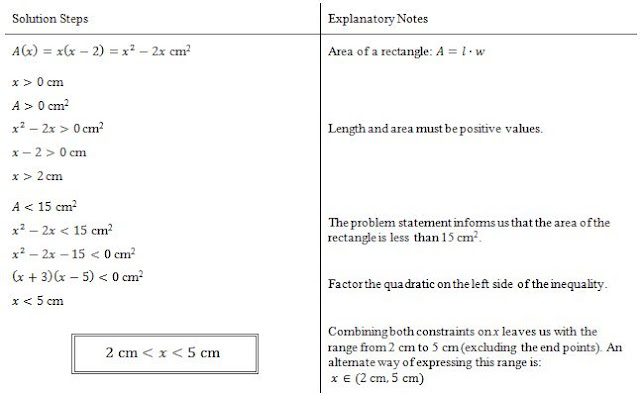Solution to Problem 8

Problem 9

This one could cause a few hangups for students. The question asks if three points lie on the same line, but doesn't give nearly enough information to plot these points on a line or prove that they lie on the same line. That's the sort of thing that can stick in a student's brain as they waste time agonizing over how to prove these points are collinear. The trick here is that you do have enough information to do a test that could prove the three points are NOT collinear. If the slope from A to B is not the same as the slope from B to C, you're done, the points can't possibly be on the same line. The slope from A to B is the same as the slope of the line given by the equation in the problem statement. The slope from B to C is a little less straightforward because you'd have to remember your trigonometric ratios (e.g. from the unit circle).Solution to Problem 9

Problem 10

I thought this one was pretty easy, but I can see this potentially being a colossal waste of time for students that didn't recognize how to attack the problem. Students had to remember the 3-4-5 triangle, or at least what "soh cah toa" meant and then put it to use. That's enough for part (a). Part (b) required some algebraic manipulation of a trig identity. Several identities were provided on the formulae list included with the test, so the student would've needed to decide which one would actually help them solve the problem. A bad choice would've led to a fruitless algebra exercise, costing precious time.Solution to Problem 10

Problem 11

In terms of marks, this question was the most important one of the test. You had to find the tangent to the circle. You could have used implicit differentiation here to get the slope of the tangent at T, but I'm not sure that's taught in high schools these days. I solved part (a) without using calculus. If the student did not know how to use implicit differentiation, the student had to recall that any line from the centre of a circle to a point on the circumference is perpendicular to the circle, and that two lines are perpendicular to each other if one slope is equal to the negative reciprocal of the other slope. For part (b) the student had to take the first derivative to find the tangent to the parabola and then solve for the value of p that makes the tangent to the parabola equal to the tangent from part (a).Solution to Problem 11

Problem 12

Based on the symmetry of a harmonic function like y = acos bx, you might recognize that the unshaded area is 2 times the shaded area, and that the unshaded area would be a negative contribution to the integral because it is below the x-axis. If you did that, you'd have this solved in a just a few seconds. But you could also do this the hard way by solving for a and b and then solving the integral. The latter approach could be a big waste of time, though a well-prepared student probably could still solve it in 2 to 3 minutes, which is pretty close to the 70 seconds per mark you need to average to finish the test on time.Solution to Problem 12

Problem 13

This is the question you were looking for: the problem I didn't solve... within the time allotment. Specifically, it was part (c) that got me. Parts (a) and (b(i)) were no problem. I was confused about what they meant by "images" of P and Q in (b(ii)) but I correctly assumed they meant the points P' and Q' on the inverse function corresponding to points P and Q. I had no idea what they were asking about with Part (c) though so I skipped it. The answer came to me two days later. They were simply asking about function transformations, but they didn't explicitly tell you that in the problem statement. It was merely implied by referring to the images of P, Q, and R. y = 4 - f(x+1) is the transformed version of y = f(x) and the student had to provide the coordinates of the image of point R. Put another way, the student had state where point R would map to after undergoing the same transformations that turn y = f(x) into y = 4 - f(x+1).Solution to Problem 13

Problem 14

I thought this was an interesting question because you had to think a little bit about how a circle could intersect the axes at exactly three points, and there are two correct answers to this problem. One possibility is that the circle just touches one axis and intersects the other axis at two points. The other possibility is that the circle intersects the axes at the point (0,0) with an additional intersection point on each of the axes. In either case, you start by finding the centre of the circle, which is pretty straightforward because that information can be extracted from the equation. If the circle intersects the origin, set k = 0 and solve for the radius (equal to the distance from the origin to the centre of the circle). If the circle touches one axis but intersects the other at two points, the set the radius to whichever centre co-ordinate has larger magnitude (in this problem it was the x-coordinate), then solve for k.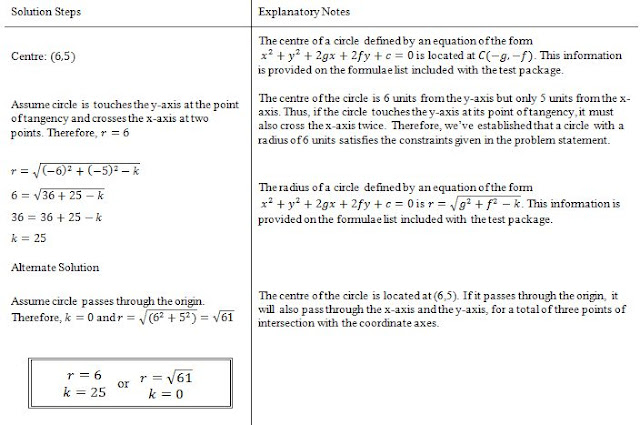Solution to Problem 14

Problem 15Here the student is given an expression for the rate of change of the temperature of some coffee and is asked to find an expression for the temperature versus time. This problem requires an integration. It's a fairly simple integration since it's just a linear function. An important thing to remember here is to include the integration constant and then solve for it using the boundary conditions provided in the problem statement.

 Solution to Problem 15

In summary, Part One of the 2015 SQA Higher Mathematics Examination didn't have any questions that were especially difficult to solve using high school math skills. However, the time limit likely hurt a lot of students. Students needed to quickly recognize an efficient way to solve each problem and work through the solutions with confidence. Excluding 13 (c), I finished with time to spare, though not as much spare time as I expected. On top of the amount of time to read the questions and go through the process of calculating and writing the solutions, students likely had a relatively small cushion of time for any pauses to think about the problems, review solutions, or second-guess themselves. To do really well on this exam you definitely had to know your stuff well and at times demonstrate a little ingenuity to find the fastest route to the answer. These are reasonable expectations for a math exam (after all, if the test was too easy, it'd be difficult to distinguish the most brilliant students from the average). All that being said, because the questions weren't all that difficult, I believe the average student should've at least been able to earn an honest pass with a grade above 50%.